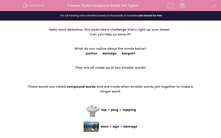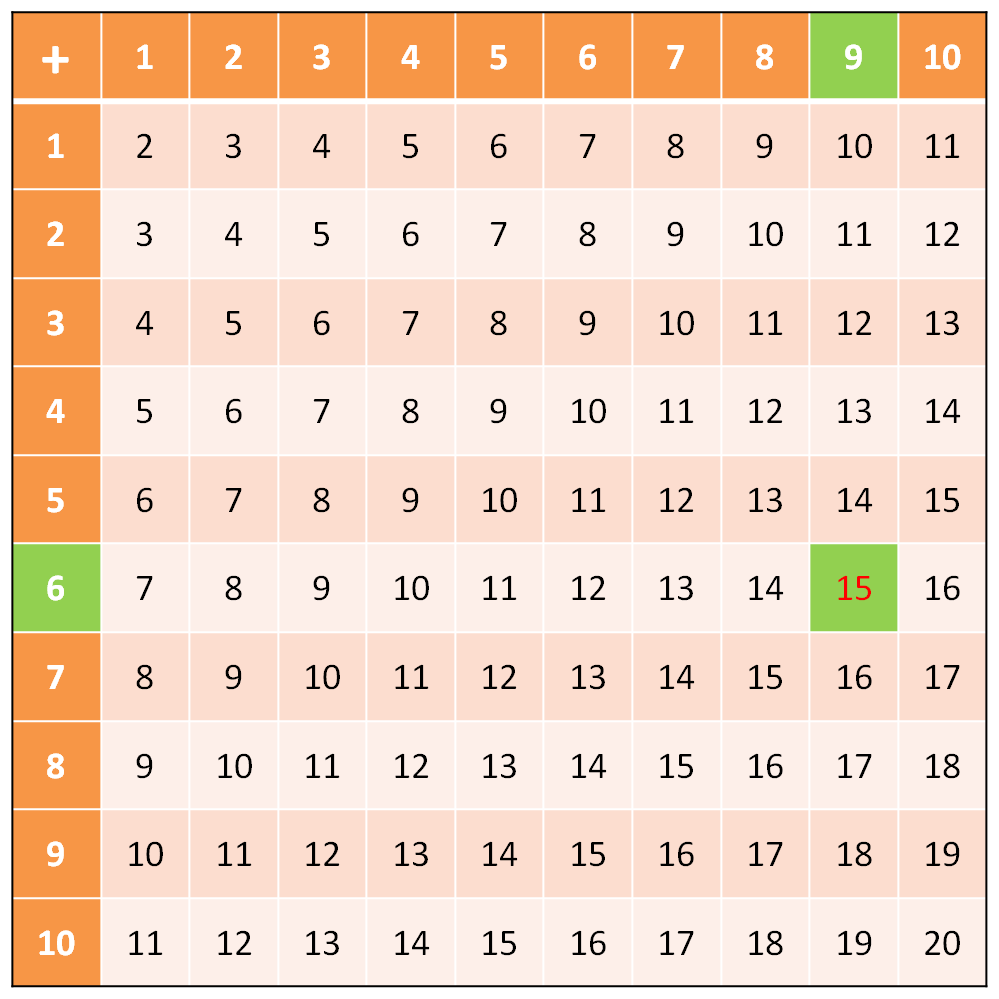# Practise Using Addition and Subtraction Facts to 100

In this worksheet, students will practise their addition and subtraction number bonds to 20 and use them to derive related facts to 100.Key stage:  KS 1

Curriculum topic:   Number: Addition and Subtraction

Curriculum subtopic:   Use Addition/Subtraction Facts to 100

Difficulty level:#### Worksheet Overview

Look at this number square which shows all the bonds you should know by heart up to 20.You can use this table to work out additions and subtractions right up to 100.

Example 1

Method 1:

We split 76 into 70 and 6.

We now need to work out 70 + 6 + 9

You should know this by heart, but the number square shows that 6 + 9 = 15So, 70 + 6 + 9 = 70 + 15

70 + 15 = 85

Method 2:

Can you make the calculation easier by slightly changing it?

In this example, you are adding 9. How much easier would it be if you added 10?

Let's try: 76 + 10 = 86.   Easy right?

But, we were supposed to add only 9! So, simply subtract 1 and you've got your answer: 86 - 1 = 85

Example 2

Do this subtraction:  85 - 9

Method 1:

If we subtract 5 from 85, we get 80, but we need to subtract 9. How much more do we need to subtract if we need to subtract 9 in total?

Use your number square and you will see that 9 - 5 = 4. This means that we need to subtract 4 more from 80 in order to have subtracted 9 in total.

So we can do:

85 - 5 = 80

80 - 4 = 76 (We can work this out from knowing our adding bonds to 10: 10 - 4 = 6, so 80 - 4 = 76)

Method 2:

Again, can you make the calculation easier by slightly changing it?

In this example, you are subtracting 9. How much easier would it be if you subtracted 10?

Let's try: 85 - 10 = 75

But, we were supposed to subtract 9! So, simply add 1 and you've got your answer: 75 + 1 = 76

So, 85 - 9 = 76

Can you see that there is often more than one way to work out an addition or subtraction problem? It doesn't matter which one you use - just choose the one that works best for you.

Are you ready to have a go at some questions now?

You can check back to the 100 square at any point by clicking on the red help button on  the screen.### What is EdPlace?

We're your National Curriculum aligned online education content provider helping each child succeed in English, maths and science from year 1 to GCSE. With an EdPlace account you’ll be able to track and measure progress, helping each child achieve their best. We build confidence and attainment by personalising each child’s learning at a level that suits them.

Get started